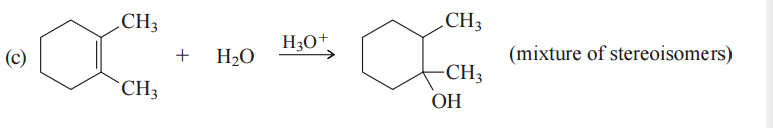Problem: Suggest reasonable mechanisms for each of the following reactions. Use curved arrows to show electron flow.

94% (41 ratings)
Problem Details

Suggest reasonable mechanisms for each of the following reactions. Use curved arrows to show electron flow.What scientific concept do you need to know in order to solve this problem?

Our tutors have indicated that to solve this problem you will need to apply the Acid-Catalyzed Hydration concept. You can view video lessons to learn Acid-Catalyzed Hydration Or if you need more Acid-Catalyzed Hydration practice, you can also practice Acid-Catalyzed Hydration practice problems .

What is the difficulty of this problem?

Our tutors rated the difficulty of Suggest reasonable mechanisms for each of the following reac... as low difficulty.

What professor is this problem relevant for?

Based on our data, we think this problem is relevant for Professor Thomas' class at UCF.

What textbook is this problem found in?

Our data indicates that this problem or a close variation was asked in . You can also practice practice problems .